# Get the first N elements of an Array in JavaScriptLast updated: Jan 5, 2023
4 min## #Get the first N elements of an Array in JavaScript

To get the first N elements of an array, call the `slice()` method passing it `0` and `N` as arguments.

For example, `arr.slice(0, 2);` returns a new array containing the first two elements of the original array.

index.js
```Copied!```const arr = ['a', 'b', 'c', 'd', 'e', 'f'];

// ✅ get first 2 elements on an array
const first2 = arr.slice(0, 2);
console.log(first2); // 👉️ ['a', 'b']

// ✅ get first 3 elements on an array
const first3 = arr.slice(0, 3);
console.log(first3); // 👉️ ['a', 'b', 'c']
``````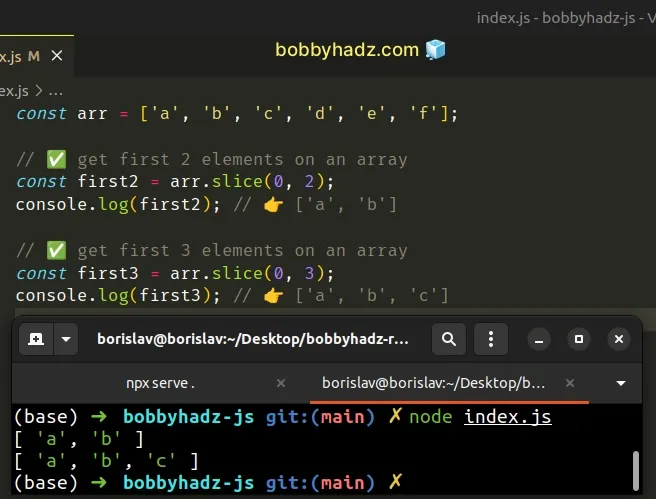The Array.slice method returns a copy of a portion of an array.

The method takes the following 2 arguments:

NameDescription
start indexThe index of the first element to include in the returned array
end indexThe index of the first element to exclude from the returned array

We used a start index of `0` to start extracting elements from the start of the array.

index.js
```Copied!```const arr = ['a', 'b', 'c', 'd', 'e', 'f'];

const first2 = arr.slice(0, 2);
console.log(first2); // 👉️ ['a', 'b']
``````

When the `start` index is `0`, you can think of the `end` index as the number of elements the new array should contain.

For example, `arr.slice(0, 3)` returns a new array containing the first 3 elements of the original array (array elements at index `0`, `1` and `2`).

The end index is exclusive, so the slice goes up to, but not including the specified end index.

If the specified end index exceeds the array's length, the `slice()` method doesn't throw an error, it returns the entire array.

index.js
```Copied!```const arr = ['a', 'b', 'c'];

const first100 = arr.slice(0, 100);
console.log(first100); // 👉️ ['a', 'b', 'c']
``````

We tried to get the first `100` elements of an array that only contains `3` elements.

As a result, the new array contains all `3` elements of the original array.

The slice method doesn't change the contents of the original array, it returns a new array.

You can also use destructuring assignment to get the first N elements of an array.

## #Get the first N elements of an Array using destructuring

The destructuring assignment syntax enables us to assign the first N elements of an array to variables.

index.js
```Copied!```const arr = ['a', 'b', 'c', 'd', 'e', 'f'];

const [first, second] = arr;

console.log(first); // 👉️ 'a'
console.log(second); // 👉️ 'b'
``````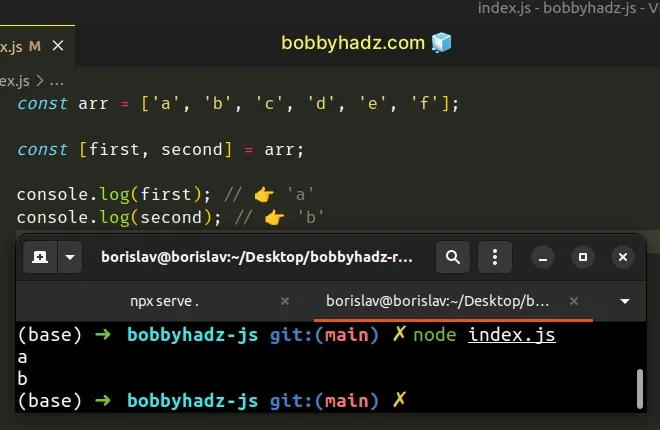The `first` and `second` variables got assigned the values of the first and second array elements.

When using destructuring, the order of variable declaration and array elements is preserved. It goes from left (element at index 0) to right (index 1), etc.

You can skip an element when destructuring by using a comma.

index.js
```Copied!```const arr = ['a', 'b', 'c', 'd', 'e', 'f'];

const [, second, third] = arr;

console.log(second); // 👉️ 'b'
console.log(third); // 👉️ 'c'
``````

We used a comma to skip the array element at index `0` and assigned the second and third array elements to variables.

You can also use a basic `for` loop.

## #Get the first N elements of an Array using a `for` loop

This is a three-step process:

1. Declare a new variable that stores an empty array.
2. Use a `for` loop to iterate for N - 1 iterations.
3. Push the element at the current index into the new array.
index.js
```Copied!```const arr = ['a', 'b', 'c', 'd', 'e', 'f'];

const newArray = [];

const n = 3;

for (let index = 0; index < n; index++) {
newArray.push(arr[index]);
}

console.log(newArray); // 👉️ [ 'a', 'b', 'c' ]
``````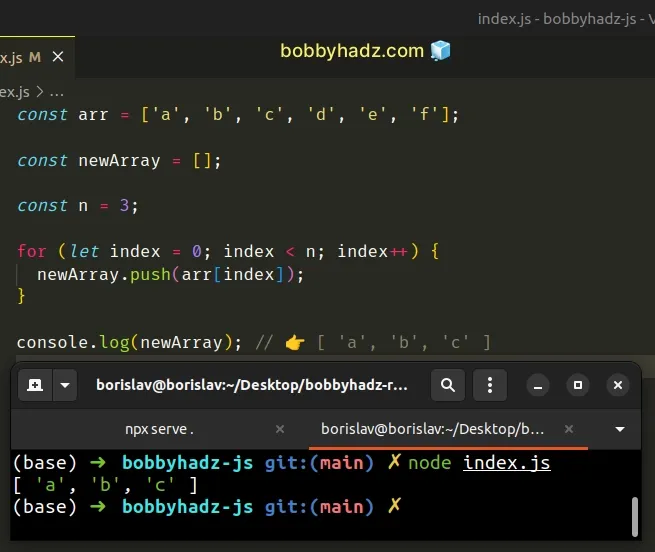We used a `for` loop to iterate for `n - 1` iterations.

On each iteration, we access the array element at the current index and push it into a new array.

The Array.push method adds an element to the end of an array.

The `for` loop only iterates for as many iterations as necessary.

You might also see the `filter()` method used to get the first N elements of an array, however, it is not a good choice.

## #Get the first N elements of an Array using `filter`

The `filter()` method is not suitable for this task because it iterates over the entire array.

index.js
```Copied!```const arr = ['a', 'b', 'c', 'd', 'e', 'f'];

const n = 3;

const first3 = arr.filter((element, index) => index < n);
console.log(first3); // 👉️ [ 'a', 'b', 'c' ]
``````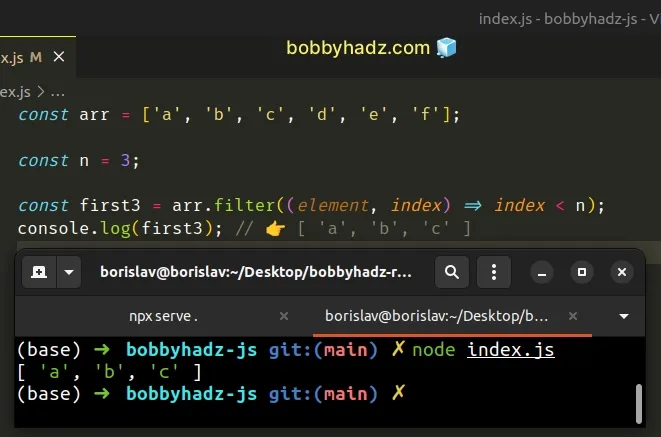The function we passed to the Array.filter method gets called with each element in the array.

On each iteration, we check if the current index is less than `n` and return the result.

The `filter()` method returns a new array that only contains the elements that meet the condition.

However, the `filter()` method is not suitable for the task because it keeps iterating over the array even after we've selected the first N elements.

This is bad for performance, especially when working with large arrays with thousands of elements.

## #Get the first N elements of an Array using `lodash`

You can also use the `take()` method from `lodash` to get the first N elements of an array.

First, make sure you have `lodash` installed by running the following command from your terminal.

shell
```Copied!```# 👇️ initialize package.json if you don't have one
npm init -y

npm install lodash
``````

Now you can import and use the take function from `lodash`.

index.js
```Copied!```import _ from 'lodash';

const arr = ['a', 'b', 'c', 'd', 'e', 'f'];

const first2 = _.take(arr, 2);
console.log(first2); // 👉️ [ 'a', 'b' ]

const first3 = _.take(arr, 3);
console.log(first3); // 👉️ [ 'a', 'b', 'c' ]
``````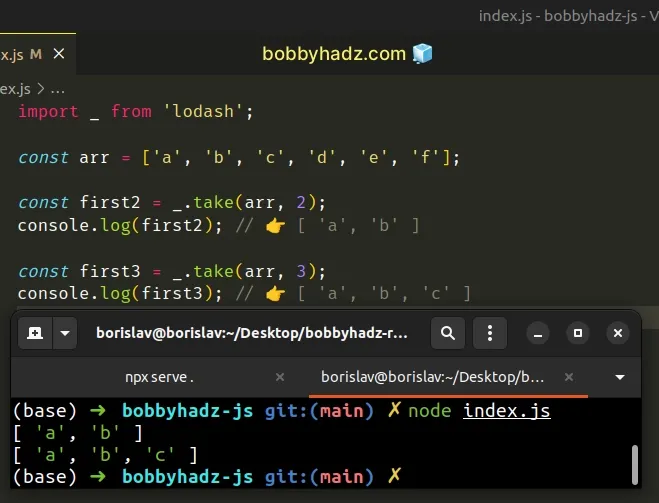The function returns a new array that contains the first `n` elements of the supplied array.

Which approach you pick is a matter of personal preference. I'd use the `Array.slice()` method as I find it quite direct and intuitive.